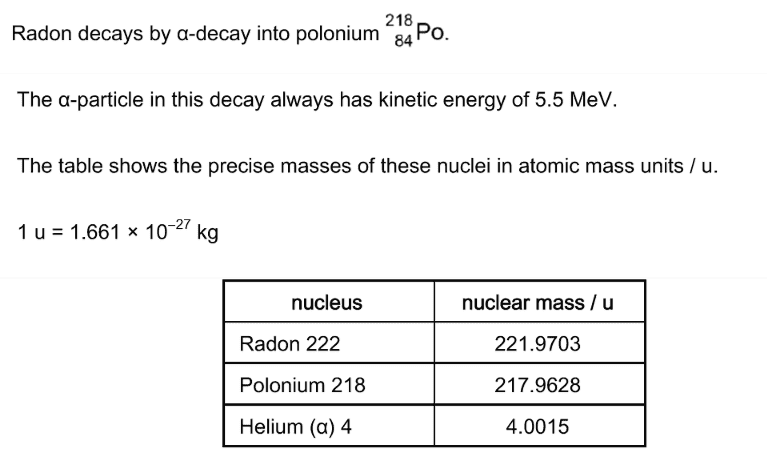# Question about final k.e. of alpha and Polonium after decay of Radon

• B
Glenn G
TL;DR Summary
Question into mass loss converted to ke alpha and Po nucleus BUT what about extra ke gained from the now repelling +charge Po and alpha particle (since not bonded by strong nuclear force now) !!! Please help
Hi community,
I've been looking through this questionAnd I have a conceptual if I may (this is not homework I'm a 52 year old programmer just trying to learn and understand).
You can calculate the mass loss of 0.006 u when you go from the Radon nucleus to the Polonium and alpha particle.
You can calculate that this equates to an energy of 5.61MeV ( so this change of mass that now has become energy has to go somewhere right? and so let's give the He and Po k.e. after the interaction)
So all this is fine the 5.61MeV is enough to give the alpha the 5.5MeV mentioned above (with 0.11MeV left over for the recoiling Po nucleus)

What then confused me was ... well hang on we now could think of the Po and He being next to each other for an instant (but no longer bound by the strong nuclear force) but NOW there is the electrostatic repulsion between the protons in the He and the Po SO wouldn't this mean that this initial 5.61MeV ke (that comes at the expense of the mass loss) that the products share starts to now increase due to the electrostatic repulsive forces SO would that mean that the final sum of the kinetic energies of the alpha and Po is LARGER than the 5.61MeV (because they've gained extra at the expense of some stored electrical potential energy)

Consequently if the alpha is recorded as having 5.5MeV at some distance from the Polonium then we can assume that the extra ke from the electrostatic repulsion has kind of pretty much happened such that the recoiled Po would have a recoil KE of bigger than 0.11MeV.

I hope I've explained my confusion OK and i would really appreciate help with this.

kind regards,

Glenn.

Gold Member
When you do conservation of mass/energy you suppose that at the final state the particles are infinitely distant so they do not feel any kind of force. This means that those 5.61MeV you got are only of kinetic origin (in the final state). If you consider the particles to be near each other they will feel an interaction. In this case each particle will have some kinetic energy and some potential energy, but the total energy difference is stil 5.61MeV. This means that the alpha particle will have a kinetic energy (slightly) lower* then 5.5MeV and will also have some potential energy to compensate for the difference.
Good question though. The interaction between charged particles is the reason for the different curves obtained from ##\beta^+## and ##\beta^-## decays (which otherwise would have been identical).
Hope I was clear

*this assuming the interaction is repulsive. The sign of the potential energy obviously depends on the nature of the interaction (repulsion/attraction)

•Glenn G
Glenn G
Hi dRic2,
That is great thanks very much, really appreciate your response - I'm glad its that. I had wondered if it is something like that as I'd considered a scenario of two protons that are fired at each other, clearly there is elec. potential energy energy increasing as they approach each other and then if they get close enough for the strong nuclear force to take hold they fuse together. Now the potential can't just disappear but must be accounted for in the interaction somehow and so if a nucleus decays at some point in time the stored elec pot energy will get converted back into k.e.
What is really cool is this idea that the initial mass loss that occurs that get converted into initial right after the separation it is in the form of ke and e.p.e but at distance just becomes ke.
Thanks again, really appreciate it.

•dRic2